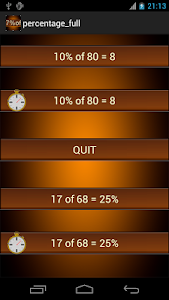# percentage math homeworks v1.8 (Paid)

Free

### percentage math homeworks / Screenshots### percentage math homeworks / Description

This program is designed to give users basic math practice with percent
calculations. Two types of math problems involving percents will be continuously
generated in a random order.

This application has four different variations of the game so players can choose
the type of percent calculations they want to practice and whether to play at their
own pace or against the clock.

(The following is from the program's menu screen)
How to play:
Make a selection from the menu for the game you want to play.

Calculate a percentage of a number (e.g. 10% of 80 = ? ). Play at your own pace.
Calculate a percentage of a number (e.g. 10% of 80 = ? ). Play against the clock.

QUIT

Calculate what percentage a number is of another number. (e.g. 17 of 68 = ?% ) Play at your own pace.
Calculate what percentage a number is of another number. (e.g. 17 of 68 = ?% ) Play against the clock.

Correct answers are shown in green; incorrect answers are shown in red.

In the play at your own pace mode: If you have the wrong answer you will see the
correct answer and how it should be calculated above the menu button.

In the play against the clock mode: You have 120 seconds to solve as many
equations as possible. After 120 seconds your score and skill level will be
displayed. The skill level rating is calculated by subtracting the player's total
number of incorrect answers from the player's total number of correct answers.
the player's skill level rating would be 22.

Skill Level Ratings

***** exceptional 28 or more
**** outstanding 24-27
*** superior 20-23
** excellent 15-19
* very good 10-14
good 5-10

To exit the game click the "MENU" button and then click "QUIT".

All calculations will be saved in a file labeled "percentage.htm" in the "math"
folder on your external memory card or internal storage.
If you are unable to open the file "percentage.htm" you may need to download
"Open in Browser"(Denis Nelubin) and "ES File Explorer".
With the saved calculations this is a great tool for giving homeworks.

[email protected]

### percentage math homeworks / What's New in v1.8 (Paid)

- @Lirico
The correct answer and how it should be calculated is shown no matter the answer is correct or wrong.